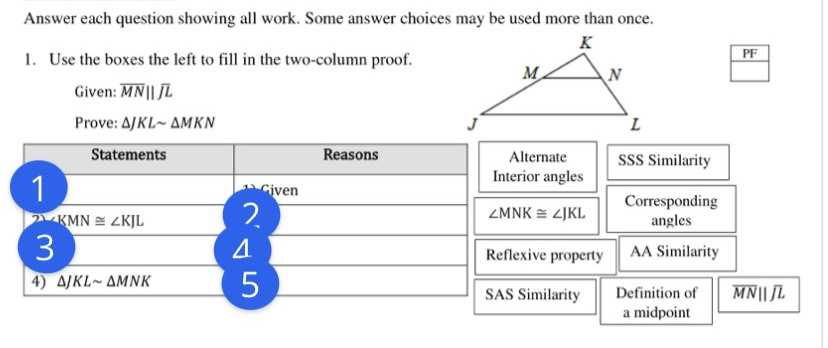### Still have math questions?

Trigonometry
QuestionAnswer each question showing all work. Some answer choices may be used more than once. 1. Use the boxes the left to fill in the two-column proof. Given: $$\overline { M N } \| \overline { J L }$$

Prove: $$\Delta J K L \sim \Delta M K N$$

1) MN $$\left | \right |$$ JL
3) $$\angle$$KNM = $$\angle$$KLJ